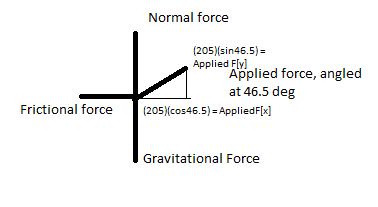# A box being pulled at an angle

## Homework Statement

A 45.0 kg box is pulled with a force of 205 N
by a rope held at an angle of 46.5° to the
horizontal. The velocity of the box increases
from 1.00 m/s to 1.50 m/s in 2.50 s. Calculate
a) Net force acting horizontally on the box
b) Frictional force on the box
c) Horizontal component of the applied force
d) Co-efficient of kinetic friction between the box and the floor

## Homework Equations

Kinematics equations
[mu][Fn] = frictional force

## The Attempt at a Solutiona) I found acceleration by: change in velocity = 0.5m/s, change in time = 2.50s
v
---
t
= 0.2m/s^2

Ok I found the summation of the X and Y forces:
b) Summation of X forces: [(205)(cos46.5) - frictionalF = (45)(0.2)]
frictionalF = 132.1N [<---]

Summation of Y forces: [Fn + (205)(sin46.5) - (45.0)(0.2) = 0]
normalF = 292.74N

c) Now, I found the horizontal component of the applied force to be:
[cos46.5] = 141.1N, but the answer at the back says 293N. Have I done something wrong?

d) I am not sure how I am going to get the coefficient.

Mg I included as (45.0)(0.2)...?

ehild
Homework Helper

Summation of Y forces: [Fn + (205)(sin46.5) - (45.0)(0.2) = 0]

The horizontal component of the applied force is 205 cos(46.5)= 141.1 N it is correct.

The coefficient of friction is obtained from
Ffr=μ Fn. Calculate Fn from the equation for the y components of forces.

ehild

Last edited:
Doesn't the applied force y-component count as one of the forces in the Y-summation?

ehild
Homework Helper
It is 205 sin(46.5) You have it already in the equation.

ehild

Ok so the back of the book is wrong. >_< Thank you.

so what did you find as fn?

I found Fn to be 292.74N, after Subtracting appliedforceY from grav.force.

isnt the normal force equal to the fappy + the force of gravity though, not fappy-fg?

Fn + Fay - Fg = 0
so
Fn = fg - Fay?

ehild
Homework Helper
I found Fn to be 292.74N, after Subtracting appliedforceY from grav.force.

It is correct. Now find the coefficient of friction.

ehild

dumb question, would the normal force be different if the object was pushed rather than pulled?

ehild
Homework Helper
Yes. The y component of the applied force would change sign.

ehild